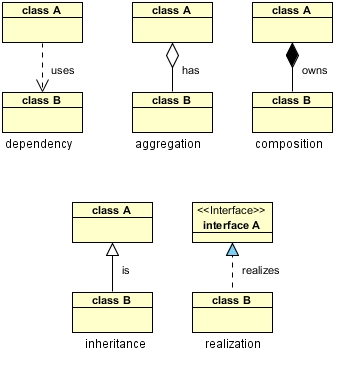UML Class Diagram Relationships, Aggregation, Composition - Tech It Yourself

Thursday, 24 June 2021

Source: here

UML Class Diagram Relationships, Aggregation, Composition

There are five key relationships between classes in a UML class diagram : dependency, aggregation, composition, inheritance and realization. These five relationships are depicted in the following diagram:UML Class Relationships
The above relationships are read as follows:
• Dependency : class A uses class B
• Aggregation : class A has a class B
• Composition : class A owns a class B
• Inheritance : class B is a Class A  (or class A is extended by class B)
• Realization : class B realizes Class A (or class A is realized by class B)
What I hope to show here is how these relationships would manifest themselves in Java so we can better understand what these relationships mean and how/when to use each one.

Dependency is represented when a reference to one class is passed in as a method parameter to another class. For example, an instance of class B is passed in to a method of class A:
public class A {

public void doSomething(B b) {

Now, if class A stored the reference to class B for later use we would have a different relationship called Aggregation. A more common and more obvious example of Aggregation would be via setter injection:
public class A {

private B _b;

public void setB(B b) { _b = b; }

Aggregation is the weaker form of object containment (one object contains other objects). The stronger form is called Composition. In Composition the containing object is responsible for the creation and life cycle of the contained object (either directly or indirectly). Following are a few examples of Composition. First, via member initialization:
public class A {

private B _b = new B();

Second, via constructor initialization:

public class A {

private B _b;

public A() {
_b = new B();
} // default constructor

Third, via lazy init (example revised 02 Mar 2014 to completely hide reference to B):

public class A {

private B _b;

public void doSomethingUniqueToB() {
if (null == _b) {
_b = new B();
}
return _b.doSomething();
} // doSomethingUniqueToB()

Inheritance is a fairly straightforward relationship to depict in Java:

public class A {

...

} // class A

public class B extends A {

....

} // class B

Realization is also straighforward in Java and deals with implementing an interface:

public interface A {

...

} // interface A

public class B implements A {

...

} // class B